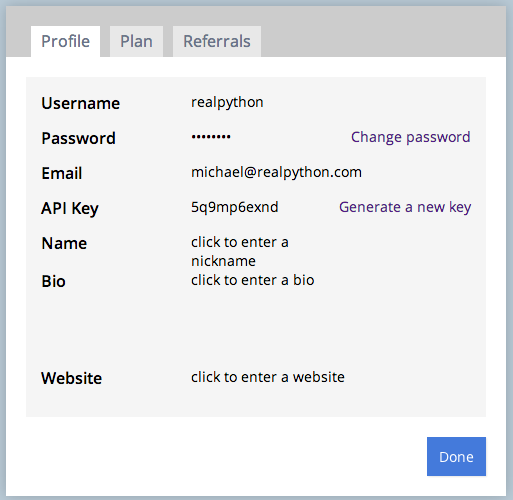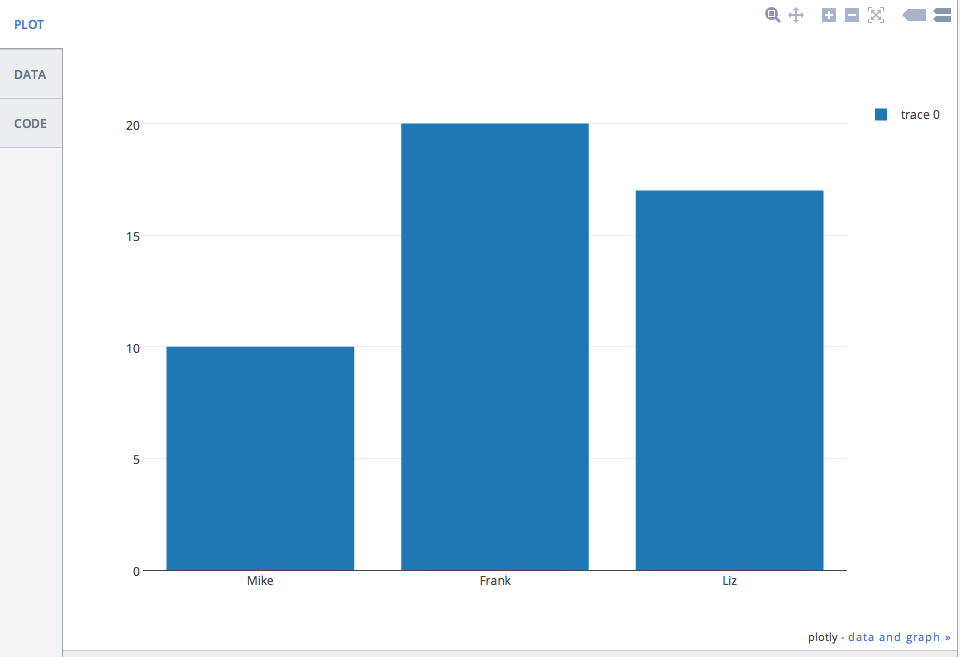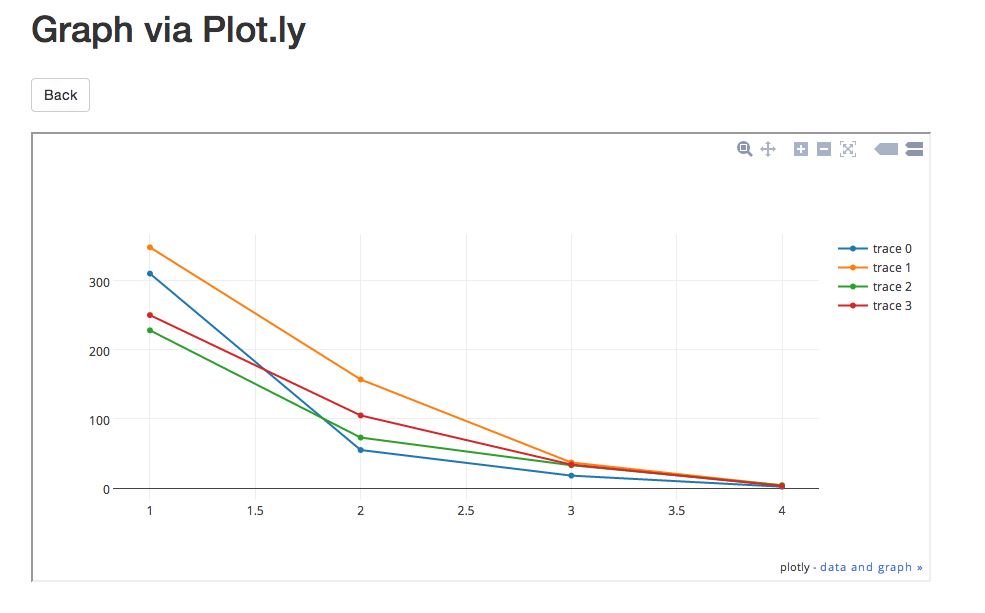# Developing with Bottle – Part 2 (plot.ly API)

Updated on 02/27/2014 and again on 08/01/2014 (for the latest Plotly API)!

In this next post in the Developing with Bottle series, we’ll be looking at both GET and POST requests as well as HTML forms. I’ll also show you how to consume data from the plot.ly API. You’ll also get to see how to create a cool graph showing the results of a cohort analysis study.

Check out this article here if you are unfamiliar with cohort analysis.

Did you miss the first part of the Bottle series? Check it out here here. Also, this tutorial uses Bottle version 0.12.3 and Plotly version 1.2.6.

## Basic Setup

```\$ bash bottle.sh
```

This will create a basic project structure:

```├── app.py
├── requirements.txt
└── testenv
```

Activate the virtualenv:

```\$ cd bottle
\$ source testenv/bin/activate
```

Install the requirements:

```\$ pip install -r requirements.txt
```Copy that key.

Install plot.ly:

```\$ pip install plotly==1.2.6
```

Next update the code in app.py:

```import os
from bottle import run, template, get, post, request

import plotly.plotly as py
from plotly.graph_objs import *

py.sign_in("realpython", "lijlflx93")

@get('/plot')
def form():
return '''<h2>Graph via Plot.ly</h2>
<form method="POST" action="/plot">
Name: <input name="name1" type="text" />
Age: <input name="age1" type="text" /><br/>
Name: <input name="name2" type="text" />
Age: <input name="age2" type="text" /><br/>
Name: <input name="name3" type="text" />
Age: <input name="age3" type="text" /><br/>
<input type="submit" />
</form>'''

@post('/plot')
def submit():
# grab data from form
name1 = request.forms.get('name1')
age1 = request.forms.get('age1')
name2 = request.forms.get('name2')
age2 = request.forms.get('age2')
name3 = request.forms.get('name3')
age3 = request.forms.get('age3')

data = Data([
Bar(
x=[name1, name2, name3],
y=[age1, age2, age3]
)
])

# make api call
response = py.plot(data, filename='basic-bar')

if response:
return template('''
<h1>Congrats!</h1>
<div>
View your graph here: <a href="{{response}}"</a>{{response}}
</div>
''',
response=response
)

if __name__ == '__main__':
port = int(os.environ.get('PORT', 8080))
run(host='0.0.0.0', port=port, debug=True)
```

### What’s going on here?

1. The first function, `form()`, creates an HTML form for capturing the data we need to make a simple bar graph.
2. Meanwhile, the second function, `submit()`, grabs the form inputs, assigns them to variables, then calls the plot.ly API, passing our credentials as well as the data, to generate a new chart. Make sure you replace my username and API key with your own credentials. DO NOT use mine. It will not work.

### Test

Run your app locally, `python app.py`, and point your browser to http://localhost:8080/plot.

Enter the names of three people and their respective ages. Press submit, and then if all is well you should see a congrats message and a URL. Click the URL to view your graph:If you get a 500 error with this message - `Aw, snap! Looks like you supplied the wrong API key. Want to try again? You can always view your key at https://plot.ly/api/key. When you display your key at https://plot.ly/api/key, make sure that you're logged in as realpython.` - you need to update your API key.

Also, if this were a real, client-facing app, you would want to handle errors much, MUCH more gracefully than this. Just FYI.

## Cohort Analysis

Next, let’s look at a more complicated example to create a graph for the following cohort analysis stats:

Cohort 2011 2012 2013 2014
0 310 348 228 250
1 55 157 73 34
2 18 37 33 34
3 2 4 4 3

We’ll be building off of the same app - app.py, but create a new file: Open app.py then “Save As” cohort.py.

Start by upgrading to the Simple Template Engine, so we can add styles and Javascript files to our templates. Add a new folder called “views” then create a new file in that directory called template.tpl. Add the following code to that file:

```<!DOCTYPE html>
<html>
<meta charset="utf-8">
<title>{{ title }}</title>
<meta name="viewport" content="width=device-width, initial-scale=1.0">
<style>
body {
}
</style>
<body>
<div class="container">
<h1>Graph via Plot.ly</h1>
<form role="form" method="post" action="/plot">
<table>
<td>
<h3>2011</h3>
<div class="form-group" "col-md-2">
<input type="number" name="Y01" class="form-control" placeholder="Cohort 0">
<input type="number" name="Y02" class="form-control" placeholder="Cohort 1">
<input type="number" name="Y03" class="form-control" placeholder="Cohort 2">
<input type="number" name="Y04" class="form-control" placeholder="Cohort 3">
</div>
</td>
<td>
<h3>2012</h3>
<div class="form-group" "col-md-2">
<input type="number" name="Y11" class="form-control" placeholder="Cohort 0">
<input type="number" name="Y12" class="form-control" placeholder="Cohort 1">
<input type="number" name="Y13" class="form-control" placeholder="Cohort 2">
<input type="number" name="Y44" class="form-control" placeholder="Cohort 3">
</div>
</td>
<td>
<h3>2013</h3>
<div class="form-group" "col-md-2">
<input type="number" name="Y21" class="form-control" placeholder="Cohort 0">
<input type="number" name="Y22" class="form-control" placeholder="Cohort 1">
<input type="number" name="Y23" class="form-control" placeholder="Cohort 2">
<input type="number" name="Y24" class="form-control" placeholder="Cohort 3">
</div>
</td>
<td>
<h3>2014</h3>
<div class="form-group" "col-md-2">
<input type="number" name="Y31" class="form-control" placeholder="Cohort 0">
<input type="number" name="Y32" class="form-control" placeholder="Cohort 1">
<input type="number" name="Y33" class="form-control" placeholder="Cohort 2">
<input type="number" name="Y34" class="form-control" placeholder="Cohort 3">
</div>
</td>
</tr>
</table>
<button type="submit" class="btn btn-default">Submit</button>
</form>
</div>
<script src="http://code.jquery.com/jquery-1.10.2.min.js"></script>
<script src="http://netdna.bootstrapcdn.com/bootstrap/3.0.0/js/bootstrap.min.js"></script>
</body>
</html>
```

As you can probably tell, this looks just like an HTML file. The difference is that we can pass Python variables to the file using the syntax - `{{ python_variable }}`.

Create a data.json file and add your Plot.ly username and API key. You can view the sample file here.

Add the following code to cohort.py in order to access the data.json to use the credentials when we make the API call:

```import os
from bottle import run, template, get, post, request

import plotly.plotly as py
from plotly.graph_objs import *

import json

# grab username and key from config/data file
with open('data.json') as config_file:
key = config_data["key"]

```

Now we don’t have to expose our key to the entire universe. Just make sure to keep it out of version control.

Next update the functions:

```import os
from bottle import run, template, get, post, request

import plotly.plotly as py
from plotly.graph_objs import *

import json

# grab username and key from config/data file
with open('data.json') as config_file:
key = config_data["key"]

@get('/plot')
def form():
return template('template', title='Plot.ly Graph')

@post('/plot')
def submit():
# grab data from form
Y01 = request.forms.get('Y01')
Y02 = request.forms.get('Y02')
Y03 = request.forms.get('Y03')
Y04 = request.forms.get('Y04')
Y11 = request.forms.get('Y11')
Y12 = request.forms.get('Y12')
Y13 = request.forms.get('Y13')
Y14 = request.forms.get('Y14')
Y21 = request.forms.get('Y21')
Y22 = request.forms.get('Y22')
Y23 = request.forms.get('Y23')
Y24 = request.forms.get('Y24')
Y31 = request.forms.get('Y31')
Y32 = request.forms.get('Y32')
Y33 = request.forms.get('Y33')
Y34 = request.forms.get('Y34')

trace1 = Scatter(
x=[1, 2, 3, 4],
y=[Y01, Y02, Y03, Y04]
)
trace2 = Scatter(
x=[1, 2, 3, 4],
y=[Y11, Y12, Y13, Y14]
)
trace3 = Scatter(
x=[1, 2, 3, 4],
y=[Y21, Y22, Y23, Y24]
)
trace4 = Scatter(
x=[1, 2, 3, 4],
y=[Y31, Y32, Y33, Y34]
)

data = Data([trace1, trace2, trace3, trace4])

# api call
plot_url = py.plot(data, filename='basic-line')

return template('template2', title='Plot.ly Graph', plot_url=str(plot_url))

if __name__ == '__main__':
port = int(os.environ.get('PORT', 8080))
run(host='0.0.0.0', port=port, debug=True)
```

Notice the `return` statement. We’re passing in the name of the template, plus any variables. Let’s create that new template called template2.tpl:

```<!DOCTYPE html>
<html>
<meta charset="utf-8">
<title>{{ title }}</title>
<meta name="viewport" content="width=device-width, initial-scale=1.0">
<style>
body {
}
</style>
<body>
<div class="container">
<h1>Graph via Plot.ly</h1>
<br>
<a href="/plot"><button class="btn btn-default">Back</button></a>
<br><br>
<iframe id="igraph" src={{plot_url}} width="900" height="450" seamless="seamless" scrolling="no"></iframe>
</div>
<script src="http://code.jquery.com/jquery-1.10.2.min.js"></script>
<script src="http://netdna.bootstrapcdn.com/bootstrap/3.0.0/js/bootstrap.min.js"></script>
</body>
</html>
```

So the iframe allows us to update the form then display the actual content/chart, with the updated changes. We do not have to leave the site to view the graph, in other words.

Run it. Add values to the form. Then submit. Your graph should now look like this:## Conclusion

You can grab all the files from this repo.

See you next time!

🐍 Python Tricks 💌

Get a short & sweet Python Trick delivered to your inbox every couple of days. No spam ever. Unsubscribe any time. Curated by the Real Python team.What Do You Think?

Real Python Comment Policy: The most useful comments are those written with the goal of learning from or helping out other readers—after reading the whole article and all the earlier comments. Complaints and insults generally won’t make the cut here.

What’s your #1 takeaway or favorite thing you learned? How are you going to put your newfound skills to use? Leave a comment below and let us know.

Keep Learning

Related Tutorial Categories: data-science web-dev